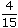# Aptitude - Ratio and Proportion - Discussion

Discussion Forum : Ratio and Proportion - General Questions (Q.No. 1)
1.
A and B together have Rs. 1210. Ifof A's amount is equal toof B's amount, how much amount does B have?
Rs. 460
Rs. 484
Rs. 550
Rs. 664
Explanation:

 4 A = 2 B 15 5A =2 x 15B 5 4A = 3 B 2A = 3 B 2A : B = 3 : 2.B's share = Rs.1210 x 2= Rs. 484. 5

Discussion:
142 comments Page 1 of 15.

Ragavan said:   2 months ago
Wonderful explanation. Thanks.
(9)

Mouni said:   4 months ago
Thank you for explaining this.
(8)

Rudraksh said:   4 months ago
Nice, Thanks everyone for the explanation.
(5)

GOBINDA BEHERA said:   4 months ago
Excellent explanation. Thanks all.
(6)

Violee said:   5 months ago
How did you get 1210? Please explain.
(6)

Thanks @Anjana.
(6)

@Sridhar.

I don't understand how it becomes 2a=3b.

(1)

Anjana Kesarwani said:   6 months ago
A4/15 = B2/5.
A/B = 3/2,
5 = 1210,
1 = 242,
B = 242 of 2 = 484.
(5)

Prashanth M said:   8 months ago
Thanks for the wonderful explanation.

Jyoti said:   1 year ago
Listen @Yogesh.

Take A=3x and B=2x.
5x= 1210 => x= 242.
Then B= 2 * 242= 484 Ans.
(12)